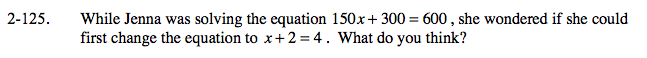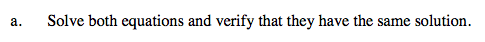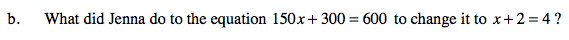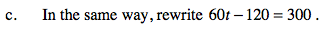### Home > CAAC > Chapter 13 > Lesson 13.RE1-S > Problem2-125

2-125.
1. While Jenna was solving the equation 150x + 300 = 600, she wondered if she could first change the equation to x + 2 = 4. What do you think? Homework Help ✎

1. Solve both equations and verify that they have the same solution.

2. What did Jenna do to the equation 150x + 300 = 600 to change it to x + 2 = 4?

3. In the same way, rewrite 60t − 120 = 300.Are the expressions on both sides of the equation divisible by 150?See part (b).